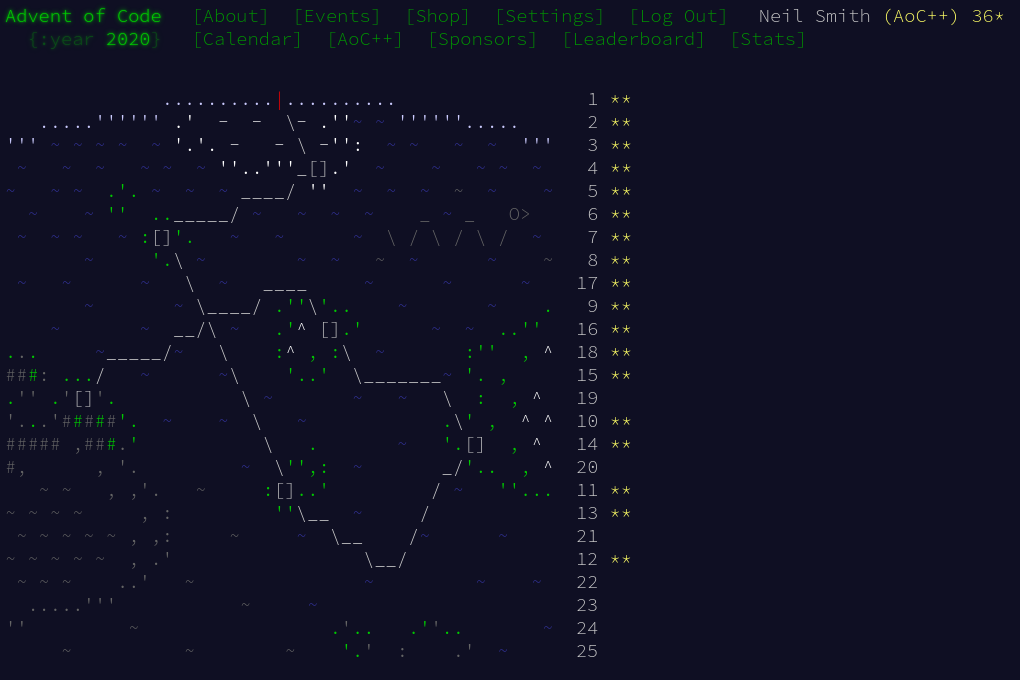December 27, 2020

# Advent of Code 2020 day 18

Going back to the source for parser combinatorsDay 18 was all about the parsing, rather than the coding. It also meant going back to the original papers that prompted the development of attoparsec and other parsing libraries.

## Data structures

This is about representing arithmetic expressions then evaluating them. As I didn't know what part 2 would bring, I thought I'd develop a data structure to hold a parsed expression, then evaluate it in a separate stage. It's just an algebraic type to hold the different operators. There's no need for a "bracket" option, as the final expression precedence is given by the shape of the Expression tree.

data Expression
= Number Integer
| Mul Expression Expression
deriving (Eq)

instance Show Expression where
show (Number x) = show x
show (Mul a b) = "(" ++ show a ++ " * " ++ show b ++ ")"
show (Add a b) = "(" ++ show a ++ " + " ++ show b ++ ")"

evaluate (Number x)  = x
evaluate (Mul a b) = (evaluate a) * (evaluate b)
evaluate (Add a b) = (evaluate a) + (evaluate b)


(In my code, I decided not to use a custom Show instance, as I wanted to be clear what the parsers were generating.

## First attempts

The parsing and association rules need to turn an expression like 1 + 2 * 3 + 4 into an Expression tree like this:

      +
/ \
*   4
/ \
+   3
/ \
1   2


A first attempt at parsing looked like this.

expressionP = mulExpression <|> addExpression
mulExpressionP = Mul <$> expressionP <* " * " *> elementP addExpressionP = Add <$> expressionP <* " + " *> elementP

elementP = numberP <|> bracketedP
bracketedP = "(" *> exprP <* ")"


Which looks good, but fails instantly with an infinite loop. A little thought reveals why: the first + needs to be a few levels down in the tree. The parser needs to parse the whole expression in order to know how many levels down it needs to be. But the structure of the parser means it needs to make a decision now about this first +, before it's seen the rest of the expression. So it keeps creating more layers in the tree, hoping they'll be enough but never terminating.

## Getting parsing working

This problem was solved in the original paper that introduced parser combinators. That paper illustrated the use of combinators with a calculator example, correctly parsing expressions like 1 + 2 + 3 into (1 + 2) + 3, which is what we want.

(Hutton, G., Meijer,  E. (1998) “Monadic parsing in Haskell,” Journal of Functional Programming 8(4): 437–444).

The trick is to use the chainl1 combinator to chain, from the left, a bunch of operations. It's easier to use a more Applicative style for this combinator (Applicative hadn't been identified in 1998!), as described by Vibhav Sagar and Stephen Diehl. I'm slightly prefer Diehl's verison, so I'm using it here.

mulExprP = string " * " *> pure Mul

expessionrP = elementP chainl1 (mulExprP <|> addExprP)

chainl1 :: Parser a -> Parser (a -> a -> a) -> Parser a
p chainl1 op = do a <- p
rest a
where rest a = (do f <- op
b <- p
rest (f a b))
<|> return a


In this instance of the chainl1 parser, it first reads an element (a number or a bracketed term) and calls it a. If it can read the op (e.g. *), it combines a and the op with the result of parsing the rest of the input. If parsing rest fails, it just returns a.

That gives us the parse we want.

(chainl1 exists in the Text.ParserCombinators.ReadP library, but its somehow missing from attoparsec. But I'm using attoparsec now, so I'll just reimplement it.)

Given the ability to parse inputs, evaluating them is easy.

main =
print $part1 text part1 = parseAndEval expressionsP parseAndEval parser text = sumEval$ successfulParse parser text

sumEval = sum . map evaluate


## Introducing precedence

Using operator precedence takes my code closer to the original Functional Pearl, even if the precedence rules are reversed from convention. All that's needed are a couple of changes to the parser, and new names to avoid confusion.

pExpressionsP = pExprP sepBy endOfLine

pExprP = pTermP chainl1 mulExprP
pTermP = pElementP chainl1 addExprP

pElementP = numberP <|> pBracketedP
pBracketedP = "(" *> pExprP <* ")"


That's all that's needed!

## Code

You can find the code here or on GitLab.